# 决策树和随机森林

### 决策树与随机森林的简单类比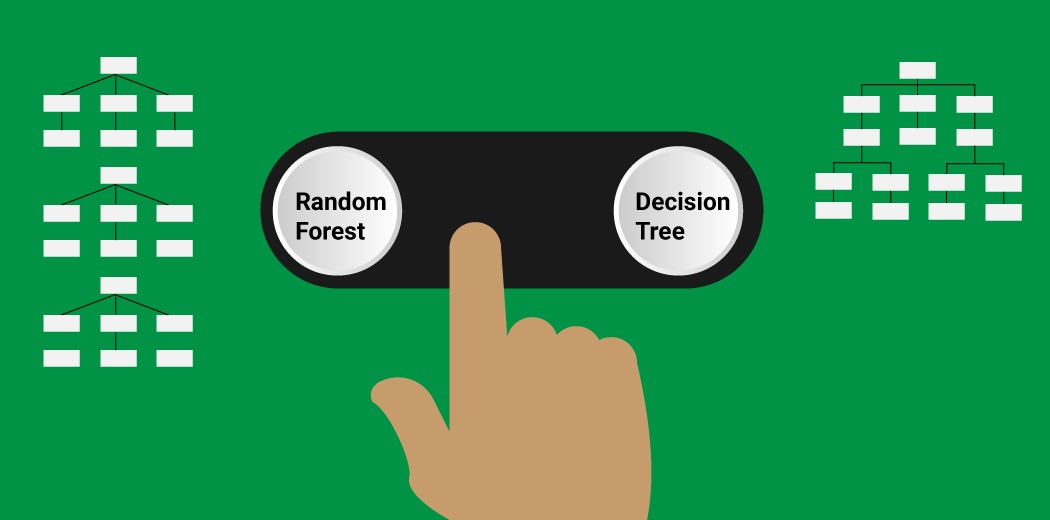### 目录

1. 决策树简介

2. 随机森林简介

3. 随机森林与决策树的冲突

4. 为什么随机森林优于决策树？

5. 决策树与随机森林—你什么时候应该选择哪种算法？

### 决策树简介### 随机森林简介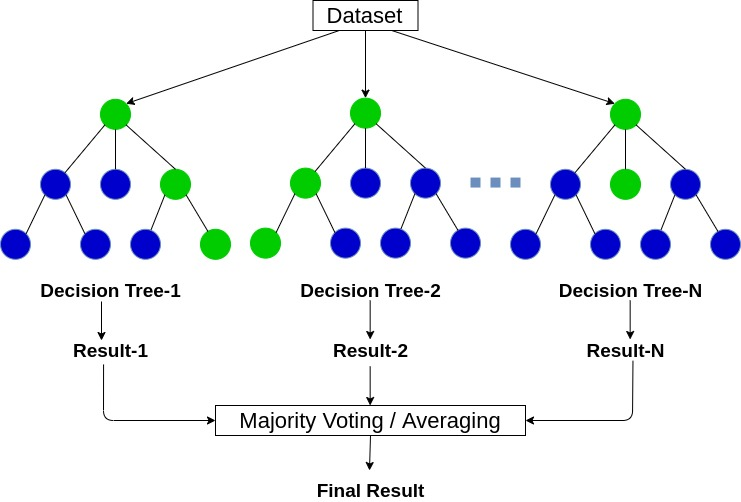### 随机森林与决策树的冲突

#### 步骤1：加载库和数据集

import pandas as pd
import numpy as np
import matplotlib.pyplot as plt
from sklearn.metrics import f1_score
from sklearn.model_selection import train_test_split

# 导入数据集#### 步骤2：数据预处理

#数据预处理与空值插补
# 标签编码
df['Gender']=df['Gender'].map({'Male':1,'Female':0})
df['Married']=df['Married'].map({'Yes':1,'No':0})
df['Dependents'].replace('3+',3,inplace=True)
df['Self_Employed']=df['Self_Employed'].map({'Yes':1,'No':0})
df['Property_Area']=df['Property_Area'].map({'Semiurban':1,'Urban':2,'Rural':3})
df['Loan_Status']=df['Loan_Status'].map({'Y':1,'N':0})

#零值插补
rev_null=['Gender','Married','Dependents','Self_Employed','Credit_History','LoanAmount','Loan_Amount_Term']
df[rev_null]=df[rev_null].replace({np.nan:df['Gender'].mode(),
np.nan:df['Married'].mode(),
np.nan:df['Dependents'].mode(),
np.nan:df['Self_Employed'].mode(),
np.nan:df['Credit_History'].mode(),
np.nan:df['LoanAmount'].mean(),
np.nan:df['Loan_Amount_Term'].mean()})#### 步骤3：创建训练和测试集

X=df.drop(columns=['Loan_ID','Loan_Status']).values
Y=df['Loan_Status'].values
X_train, X_test, Y_train, Y_test = train_test_split(X, Y, test_size = 0.2, random_state = 42)


print('Shape of X_train=>',X_train.shape)
print('Shape of X_test=>',X_test.shape)
print('Shape of Y_train=>',Y_train.shape)
print('Shape of Y_test=>',Y_test.shape)


#### 步骤4：建立和评估模型

# 建立决策树
from sklearn.tree import DecisionTreeClassifier
dt = DecisionTreeClassifier(criterion = 'entropy', random_state = 42)
dt.fit(X_train, Y_train)
dt_pred_train = dt.predict(X_train)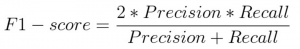#训练集评估
dt_pred_train = dt.predict(X_train)
print('Training Set Evaluation F1-Score=>',f1_score(Y_train,dt_pred_train))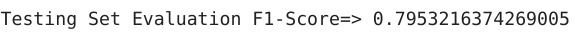#### 建立随机森林模型

# 建立随机森林分类器
from sklearn.ensemble import RandomForestClassifier
rfc = RandomForestClassifier(criterion = 'entropy', random_state = 42)
rfc.fit(X_train, Y_train)

#训练集评估
rfc_pred_train = rfc.predict(X_train)
print('Training Set Evaluation F1-Score=>',f1_score(Y_train,rfc_pred_train))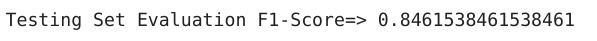### 为什么随机森林模型优于决策树？

feature_importance=pd.DataFrame({
'rfc':rfc.feature_importances_,
'dt':dt.feature_importances_
},index=df.drop(columns=['Loan_ID','Loan_Status']).columns)
feature_importance.sort_values(by='rfc',ascending=True,inplace=True)

index = np.arange(len(feature_importance))
fig, ax = plt.subplots(figsize=(18,8))
rfc_feature=ax.barh(index,feature_importance['rfc'],0.4,color='purple',label='Random Forest')
dt_feature=ax.barh(index+0.4,feature_importance['dt'],0.4,color='lightgreen',label='Decision Tree')
ax.set(yticks=index+0.4,yticklabels=feature_importance.index)

ax.legend()
plt.show()